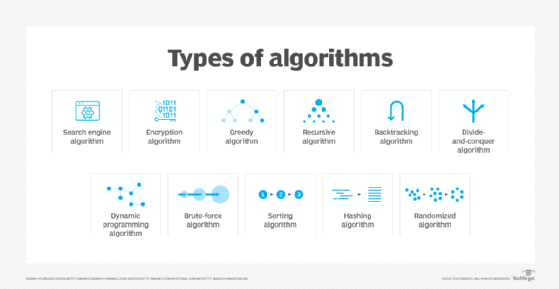×
Subscribe
Unsubscribe

# what is an algorithm

An algorithm is a procedure used for solving a problem or performing a computation. Algorithms act as a precise list of instructions that conduct specified actions step by step in either hardware- or software-based routines.

Algorithms are widely used throughout all areas of IT. In mathematics and computer science, an algorithm usually refers to a small procedure that solves a recurrent problem. Algorithms are also used as specifications for performing data processing and play a major role in automated systems.

An algorithm could be used for sorting sets of numbers or for more complicated tasks, like recommending user content on social media. Algorithms typically start with initial input and instructions that describe a specific computation. When the computation is executed, the process produces an output.

### How do algorithms work?

Algorithms can be expressed as natural languages, programming languages, pseudocode, flowcharts and control tables. Natural language expressions are rare, as they are more ambiguous. Programming languages are normally used for expressing algorithms executed by a computer.

Algorithms use an initial input along with a set of instructions. The input is the initial data needed to make decisions and can be represented in the form of numbers or words. The input data gets put through a set of instructions, or computations, which can include arithmetic and decision-making processes. The output is the last step in an algorithm and is normally expressed as more data.

For example, a search algorithm takes a search query as input and runs it through a set of instructions for searching through a database for relevant items to the query. Automation software acts as another example of algorithms, as automation follows a set of rules to complete tasks. Many algorithms make up automation software, and they all work to automate a given process.

### What are different types of algorithms?

There are several types of algorithms, all designed to accomplish different tasks. For example, algorithms perform the following:

• Search engine algorithm. This algorithm takes search stringsof keywords and operators as input, searches its associated database for relevant webpages and returns results.
• Encryption algorithm. This computing algorithm transforms data according to specified actions to protect it. A symmetric key algorithm, such as the Data Encryption Standard, for example, uses the same keyto encrypt and decrypt data. As long as the algorithm is sufficiently sophisticated, no one lacking the key can decrypt the data.
• Greedy algorithm. This algorithm solves optimization problems by finding the locally optimal solution, hoping it is the optimal solution at the global level. However, it does not guarantee the most optimal solution.
• Recursive algorithm. This algorithm calls itself repeatedly until it solves a problem. Recursive algorithms call themselves with a smaller value every time a recursive function is invoked.
• Backtracking algorithm. This algorithm finds a solution to a given problem in incremental approaches and solves it one piece at a time.
• Divide-and-conquer algorithm. This common algorithm is divided into two parts. One part divides a problem into smaller subproblems. The second part solves these problems and then combines them together to produce a solution.
• Dynamic programming algorithm. This algorithm solves problems by dividing them into subproblems. The results are then stored to be applied for future corresponding problems.
• Brute-force algorithm. This algorithm iterates all possible solutions to a problem blindly, searching for one or more solutions to a function.
• Sorting algorithm. Sorting algorithms are used to rearrange data structure based on a comparison operator, which is used to decide a new order for data.
• Hashing algorithm. This algorithm takes data and converts it into a uniform message with a hashing
• Randomized algorithm. This algorithm reduces running times and time-based complexities. It uses random elements as part of its logic.11 different types of algorithms, including search, hashing and brute force

### What are examples of algorithms?

Machine learning is a good example of an algorithm, as it uses multiple algorithms to predict outcomes without being explicitly programmed to do so. Machine learning uses supervised learning or unsupervised learning. In supervised learning, data scientists supply complex algorithms with labeled training data and define the variables they want the algorithm to assess for correlations. Both the input and the output of the algorithm are specified.

Unsupervised machine learning involves algorithms that train on unlabeled data. Unsupervised machine learning algorithms sift through unlabeled data to look for patterns that can be used to group data points into subsets. Most types of deep learning, including neural networks, are unsupervised algorithms.

Machine learning used in artificial intelligence also relies on algorithms. However, machine learning-based systems may have inherent biases in the data that feeds the machine learning algorithm. This could result in systems that are untrustworthy and potentially harmful.